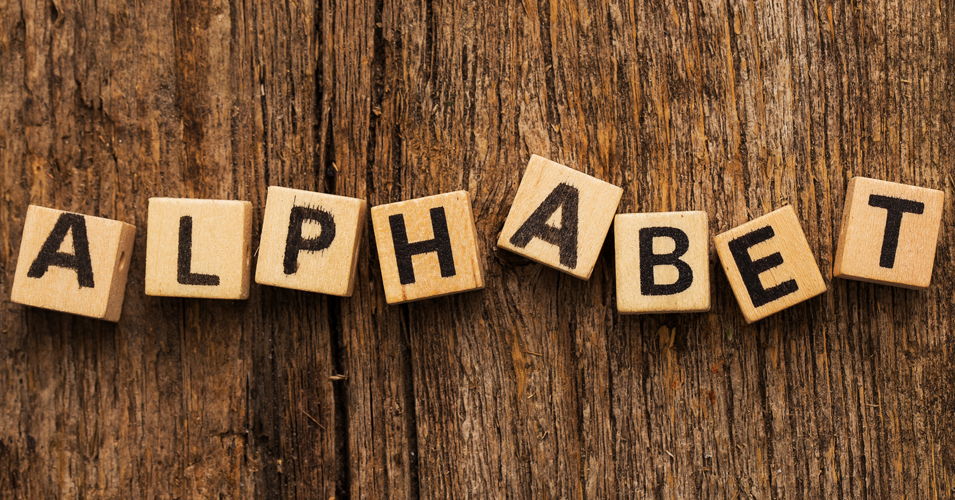• Save

# Alphabet Series Questions and Aswers for SSC and Bank ExamsAlphabet series questions are most important for competitive exams. So, don't ignore your mistakes while solving alphabet series questions in your preparation. Students should try to attempt these alphabet series questions with answers. Let's solve alphabet series questions exercise yourself for better understanding.

Preparation is the foundation of all competitive exams but it will be easy if you will do preparation with selective questions and answers. So keep practice with these Alphabet series questions and answers.

Let's solve alphabet series questions exercise yourself for better understanding with these alphabet series reasoning tricks and examples to save your time in competitive exams.

## Alphabet series questions and answers for competitive exams:

1. Which letter is seventh from left of 16th from right in English alphabet?

(A) D

(B) W

(C) R

(D) E

Ans .   A

Direction: If English alphabet is written in reverse order then-

2. Which letter is 5th at right of 12 from right?

(A) Q

(B) P

(C) G

(D) R

Ans .   C

Direction: If English alphabet is written in reverse order then-

3. If the 2nd half of an English alphabet is written in reverse order, which letter will be the 5th at left from 21st letter from left?

(A) L

(B) X

(C) M

(D) Q

Ans .   B

4. If the 2nd half of an English alphabet is written in the reverse sequence, which will be the 6th letter at right from the 18th letter from left?

(A) X

(B) P

(C) O

(D) N

Ans .   B

5. If the letters of an English alphabet are written in the reverse order, which will be the 4th letter at right from the 13th letter from left?

(A) J

(B) L

(C) K

(D) M

Ans .   A

6. Which word is at last after arranging the following according to dictionary?

(A) Satin

(B) Satire

(C) Saturn

(D) Satiate

Ans .   C

7. Arrange the following in the order of the dictionary.

1. Scenery 2. Science 3. Scandal 4. School 5. Scatter

(A) 3,5,1,4,2

(B) 3,5,4,1,2

(C) 5,3,4,2,1

(D) 5,3,2,1,4

Ans .   A

If you have any problem or doubt regarding alphabet series questions with answers, you can ask me in the comment section. To more practice for alphabet series questions with answers, Visit next page.